## Riemann Tensor

A Tensor sometimes known as the Riemann-Christoffel Tensor. Let(1)

where the quantity inside the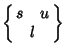is a Christoffel Symbol of the Second Kind. Then(2)

Broken down into its simplest decomposition in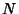-D,(3)
Here,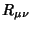is the Ricci Tensor,is the Curvature Scalar, andis the Weyl Tensor. In terms of the Jacobi Tensor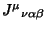,(4)

The Riemann tensor is the only tensor that can be constructed from the Metric Tensor and its first and second derivatives,(5)

whereare Connection Coefficients andare Commutation Coefficients. The number of independent coordinates in-D is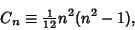(6)

and the number of Scalars which can be constructed fromandis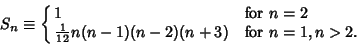(7)

In 1-D,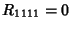.1 0 0 2 1 1 3 6 3 4 20 14

See also Bianchi Identities, Christoffel Symbol of the Second Kind, Commutation Coefficient, Connection Coefficient, Curvature Scalar, Gaussian Curvature, Jacobi Tensor, Petrov Notation, Ricci Tensor, Weyl Tensor